#Quadratic Equations: Quadratic FormulaThe Quadratic Formula. The quadratic equation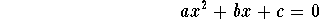has the solutions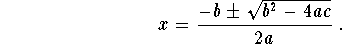with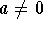. First divide both sides of the equation by a to get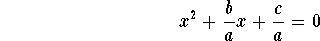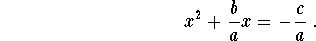Next complete the square by adding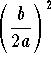to both sides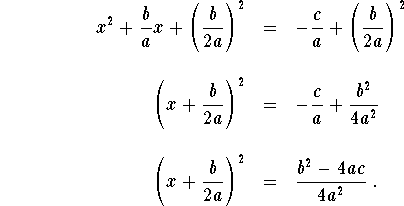Finally we take the square root of both sides: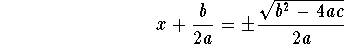or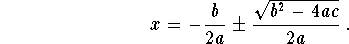We call this result the Quadratic Formula and normally write itRemark. The plus-minus sign states that you have two numbers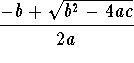and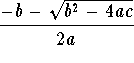.

Example: Use the Quadratic Formula to solve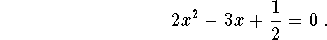Solution. We have a=2, b= -3, and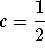. By the quadratic formula, the solutions are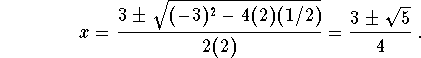Please go to General Conclusion to find a summary of all the cases regarding the roots of a quadratic equation.[Algebra] [Complex Variables]
[Geometry] [Trigonometry ]
[Calculus] [Differential Equations] [Matrix Algebra]S.O.S MATHematics home page

Do you need more help? Please post your question on our S.O.S. Mathematics CyberBoard.Author: Mohamed Amine Khamsi